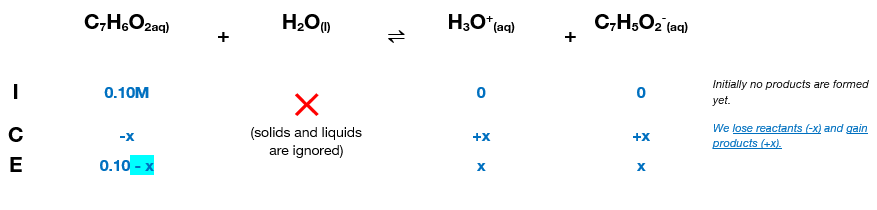# Problem: The Ka for benzoic acid is 6.5 x 10 -5. Calculate the pH of a 0.10 M benzoic acid solution

###### FREE Expert Solution

We’re being asked to calculate the pH of a 0.10 M aqueous solution of benzoic acid (C7H6O2).

Since C7H6O2 has a low Ka value, it’s a weak acid. Remember that weak acids partially dissociate in water and that acids donate H+ to the base (water in this case). The dissociation of C7H6O2 is as follows:

C7H6O2(aq) + H2O(l)  H3O+(aq) + C7H5O2 (aq); Ka = 6.5 × 10–5

From this, we can construct an ICE table. Remember that liquids are ignored in the ICE table.The Ka expression for C7H6O2 is:

Note that each concentration is raised by the stoichiometric coefficient: [C7H6O2 ], [H3O+] and [C7H5O2 ] are raised to 1.

96% (366 ratings)###### Problem Details

The Ka for benzoic acid is 6.5 x 10 -5. Calculate the pH of a 0.10 M benzoic acid solution